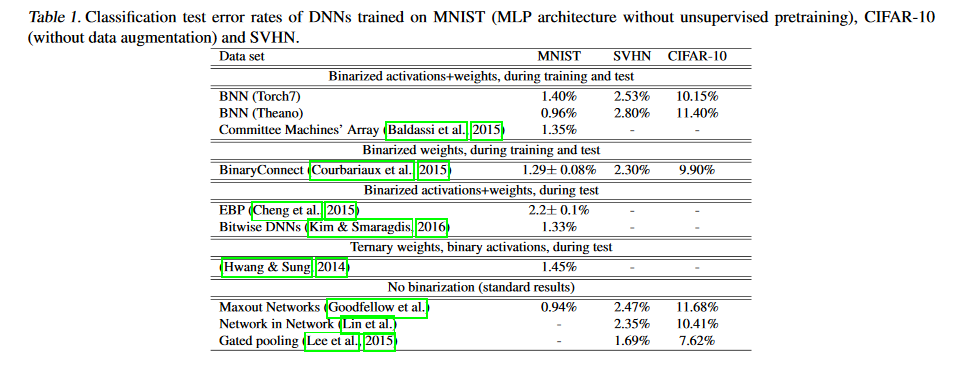# 1. 前言¶

符号位[ ] + 指数位[ ] [ ] [ ] [ ] [ ] [ ] [ ] [ ] + 尾数[ ]*23

# 2. BNN的原理¶

## 2.1 二值化方案¶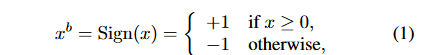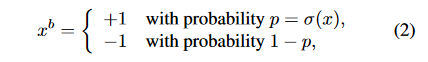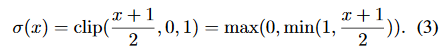## 2.2 如何反向传播？¶

$q=sign(r)$

$C$$q$的导数可以用$g_q$表示，那么$C$$r$的导数为：

$g_r=g_q1_{|r|<=1}$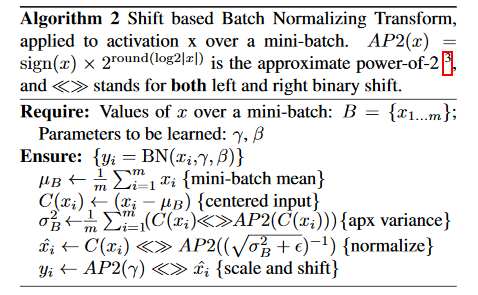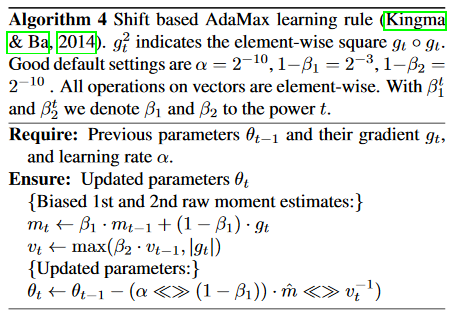## 2.3 第一层怎么办？¶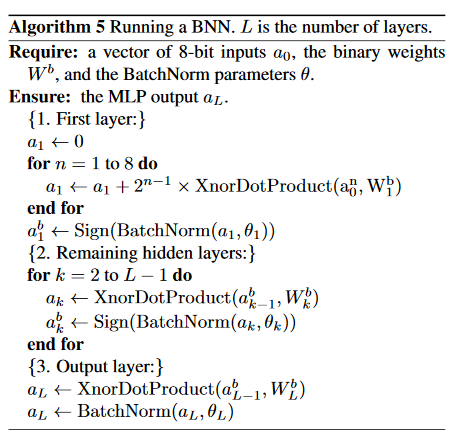$LinearQuant(x,bitwidth)=clip(round(\frac{x}{bitwidth})\times bitwidth, minV, maxV)$

$LogQuant(x,bitwidth)=clip(AP2(x), minV, maxV)$

# 3. 代码实现¶

## 3.1 定义网络结构¶

import torch.nn as nn
from .util_wt_bab import Conv2d_Q

# *********************量化(三值、二值)卷积*********************
class Tnn_Bin_Conv2d(nn.Module):
# 参数：last_relu-尾层卷积输入激活
def __init__(self, input_channels, output_channels,
kernel_size=-1, stride=-1, padding=-1, groups=1, last_relu=0, A=2, W=2):
super(Tnn_Bin_Conv2d, self).__init__()
self.A = A
self.W = W
self.last_relu = last_relu

# ********************* 量化(三/二值)卷积 *********************
self.tnn_bin_conv = Conv2d_Q(input_channels, output_channels,
self.bn = nn.BatchNorm2d(output_channels)
self.relu = nn.ReLU(inplace=True)

def forward(self, x):
x = self.tnn_bin_conv(x)
x = self.bn(x)
if self.last_relu:
x = self.relu(x)
return x

class Net(nn.Module):
def __init__(self, cfg = None, A=2, W=2):
super(Net, self).__init__()
# 模型结构与搭建
if cfg is None:
cfg = [192, 160, 96, 192, 192, 192, 192, 192]
self.tnn_bin = nn.Sequential(
nn.BatchNorm2d(cfg),
Tnn_Bin_Conv2d(cfg, cfg, kernel_size=1, stride=1, padding=0, A=A, W=W),
Tnn_Bin_Conv2d(cfg, cfg, kernel_size=1, stride=1, padding=0, A=A, W=W),

Tnn_Bin_Conv2d(cfg, cfg, kernel_size=5, stride=1, padding=2, A=A, W=W),
Tnn_Bin_Conv2d(cfg, cfg, kernel_size=1, stride=1, padding=0, A=A, W=W),
Tnn_Bin_Conv2d(cfg, cfg, kernel_size=1, stride=1, padding=0, A=A, W=W),

Tnn_Bin_Conv2d(cfg, cfg, kernel_size=3, stride=1, padding=1, A=A, W=W),
Tnn_Bin_Conv2d(cfg, cfg, kernel_size=1, stride=1, padding=0, last_relu=1, A=A, W=W),
nn.BatchNorm2d(10),
nn.ReLU(inplace=True),
)

def forward(self, x):
x = self.tnn_bin(x)
x = x.view(x.size(0), -1)
return x


## 3.2 具体实现¶

import torch
import torch.nn as nn
import torch.nn.functional as F

# ********************* 二值(+-1) ***********************
# A 对激活值进行二值化的具体实现，原理中的第一个公式
class Binary_a(Function):

@staticmethod
def forward(self, input):
self.save_for_backward(input)
output = torch.sign(input)
return output

@staticmethod
input, = self.saved_tensors
#*******************ste*********************
#****************saturate_ste***************
'''
#******************soft_ste*****************
size = input.size()
zeros = torch.zeros(size).cuda()
grad = torch.max(zeros, 1 - torch.abs(input))
'''
# W 对权重进行二值化的具体实现
class Binary_w(Function):

@staticmethod
def forward(self, input):
output = torch.sign(input)
return output

@staticmethod
#*******************ste*********************

# ********************* A(特征)量化(二值) ***********************
# 因为我们使用的网络结构不是完全的二值化，第一个卷积层是普通卷积接的ReLU激活函数，所以要判断一下
class activation_bin(nn.Module):
def __init__(self, A):
super().__init__()
self.A = A
self.relu = nn.ReLU(inplace=True)

def binary(self, input):
output = Binary_a.apply(input)
return output

def forward(self, input):
if self.A == 2:
output = self.binary(input)
# ******************** A —— 1、0 *********************
#a = torch.clamp(a, min=0)
else:
output = self.relu(input)
return output
# ********************* W(模型参数)量化(三/二值) ***********************
def meancenter_clampConvParams(w):
mean = w.data.mean(1, keepdim=True)
w.data.sub(mean) # W中心化(C方向)
w.data.clamp(-1.0, 1.0) # W截断
return w
# 对激活值进行二值化
class weight_tnn_bin(nn.Module):
def __init__(self, W):
super().__init__()
self.W = W

def binary(self, input):
output = Binary_w.apply(input)
return output

def forward(self, input):

# **************************************** W二值 *****************************************
output = meancenter_clampConvParams(input) # W中心化+截断
# **************** channel级 - E(|W|) ****************
E = torch.mean(torch.abs(output), (3, 2, 1), keepdim=True)
# **************** α(缩放因子) ****************
alpha = E
# ************** W —— +-1 **************
output = self.binary(output)
# ************** W * α **************
output = output * alpha # 若不需要α(缩放因子)，注释掉即可
# **************************************** W三值 *****************************************
else:
output = input
return output

# ********************* 量化卷积（同时量化A/W，并做卷积） ***********************
class Conv2d_Q(nn.Conv2d):
def __init__(
self,
in_channels,
out_channels,
kernel_size,
stride=1,
dilation=1,
groups=1,
bias=True,
A=2,
W=2
):
super().__init__(
in_channels=in_channels,
out_channels=out_channels,
kernel_size=kernel_size,
stride=stride,
dilation=dilation,
groups=groups,
bias=bias
)
# 实例化调用A和W量化器
self.activation_quantizer = activation_bin(A=A)
self.weight_quantizer = weight_tnn_bin(W=W)

def forward(self, input):
# 量化A和W
bin_input = self.activation_quantizer(input)
tnn_bin_weight = self.weight_quantizer(self.weight)
#print(bin_input)
#print(tnn_bin_weight)
# 用量化后的A和W做卷积
output = F.conv2d(
input=bin_input,
weight=tnn_bin_weight,
bias=self.bias,
stride=self.stride,
dilation=self.dilation,
groups=self.groups)
return output


# 4. 实验结果¶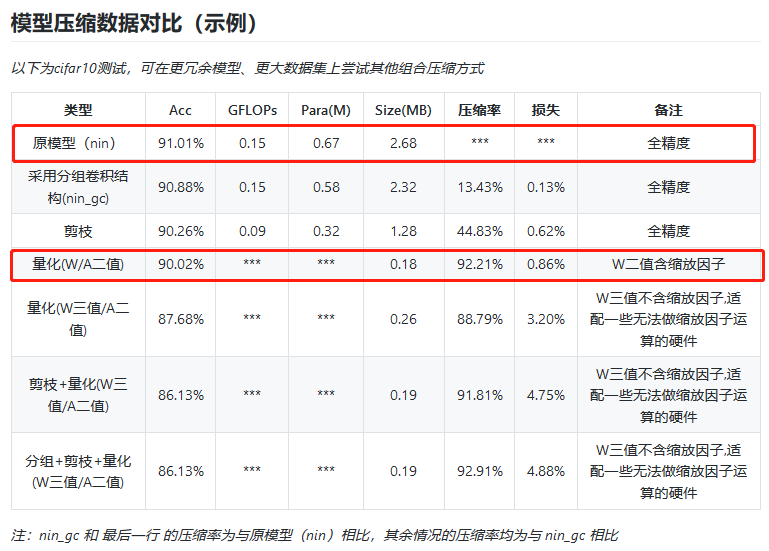# 5. 思考¶